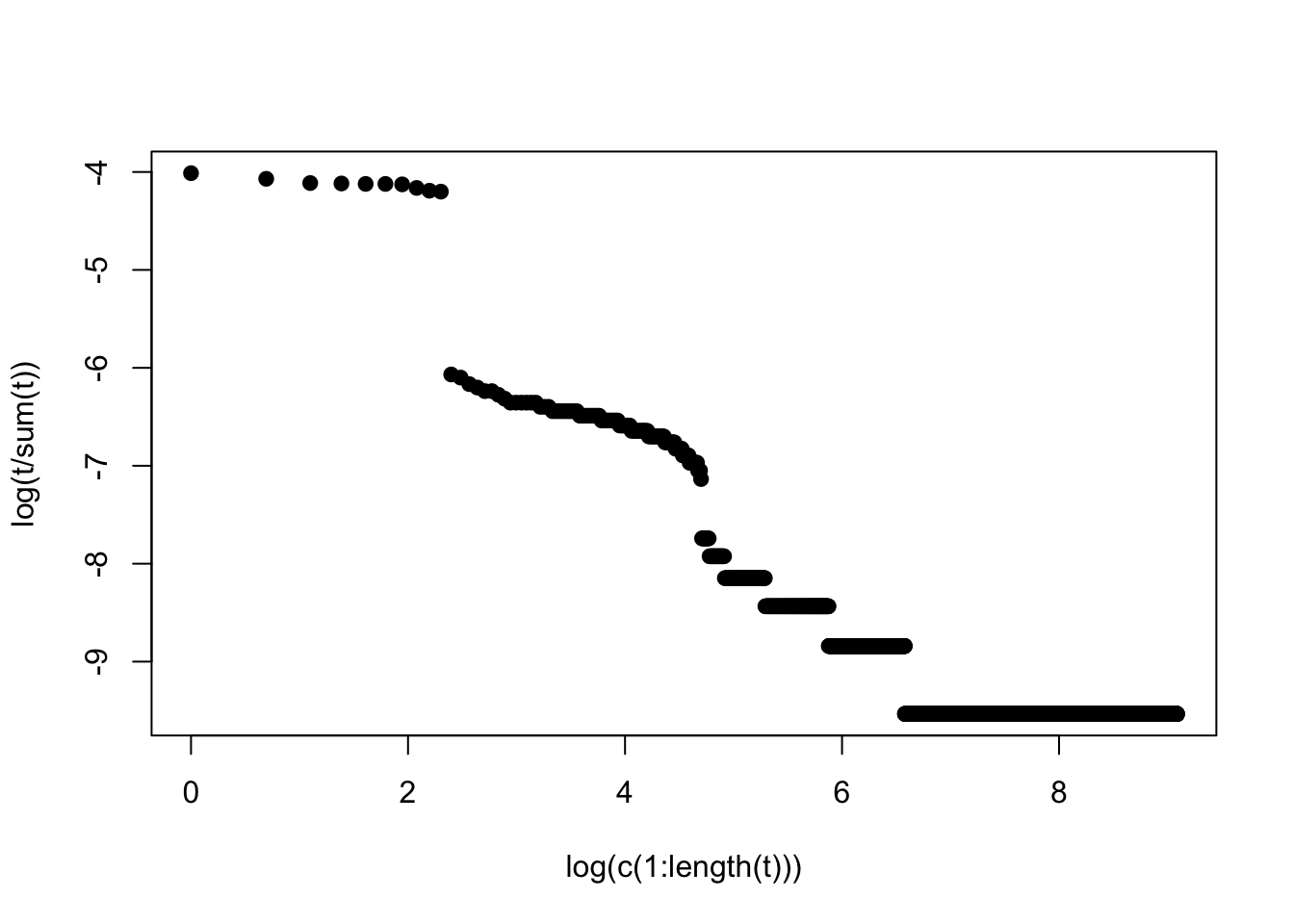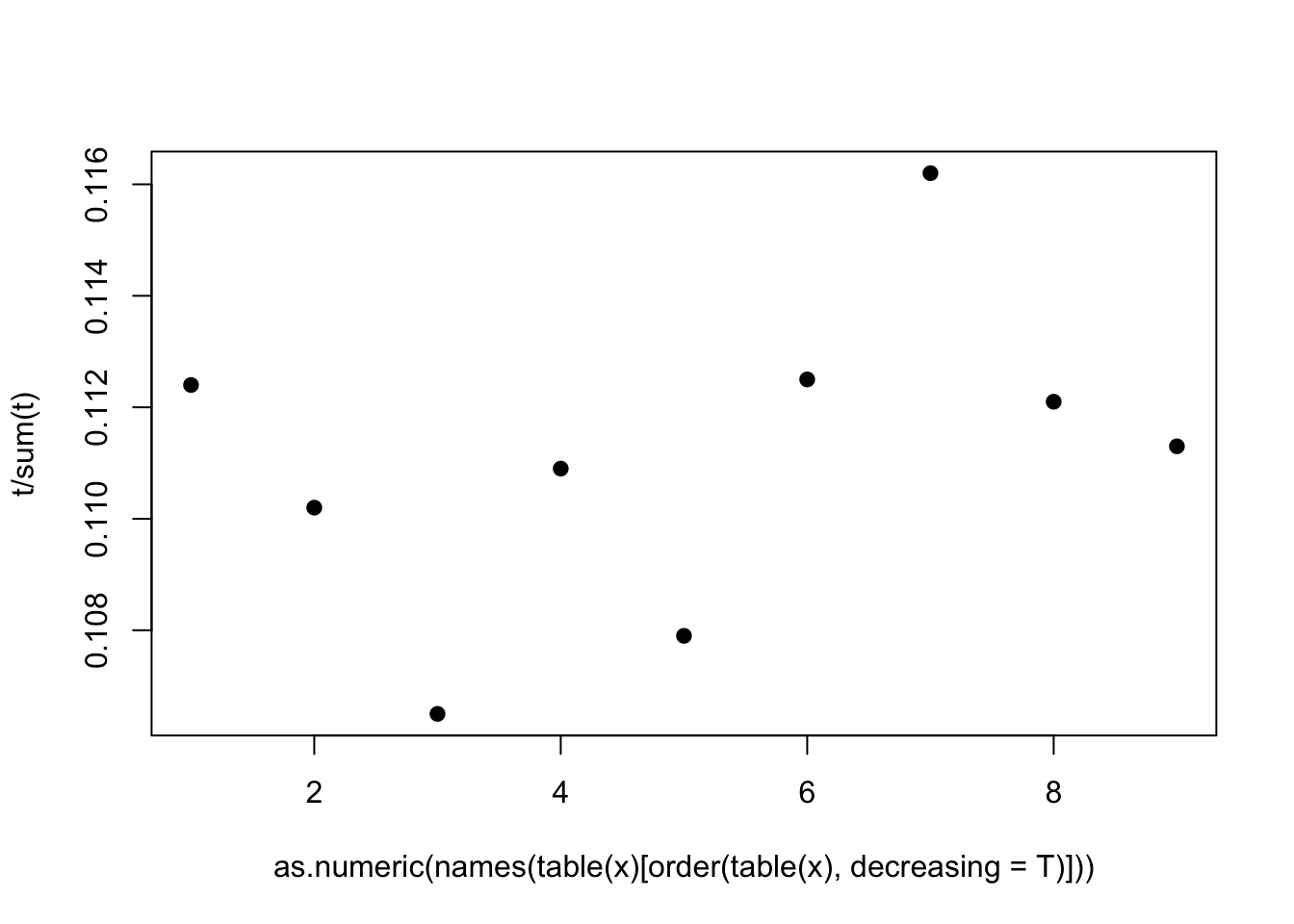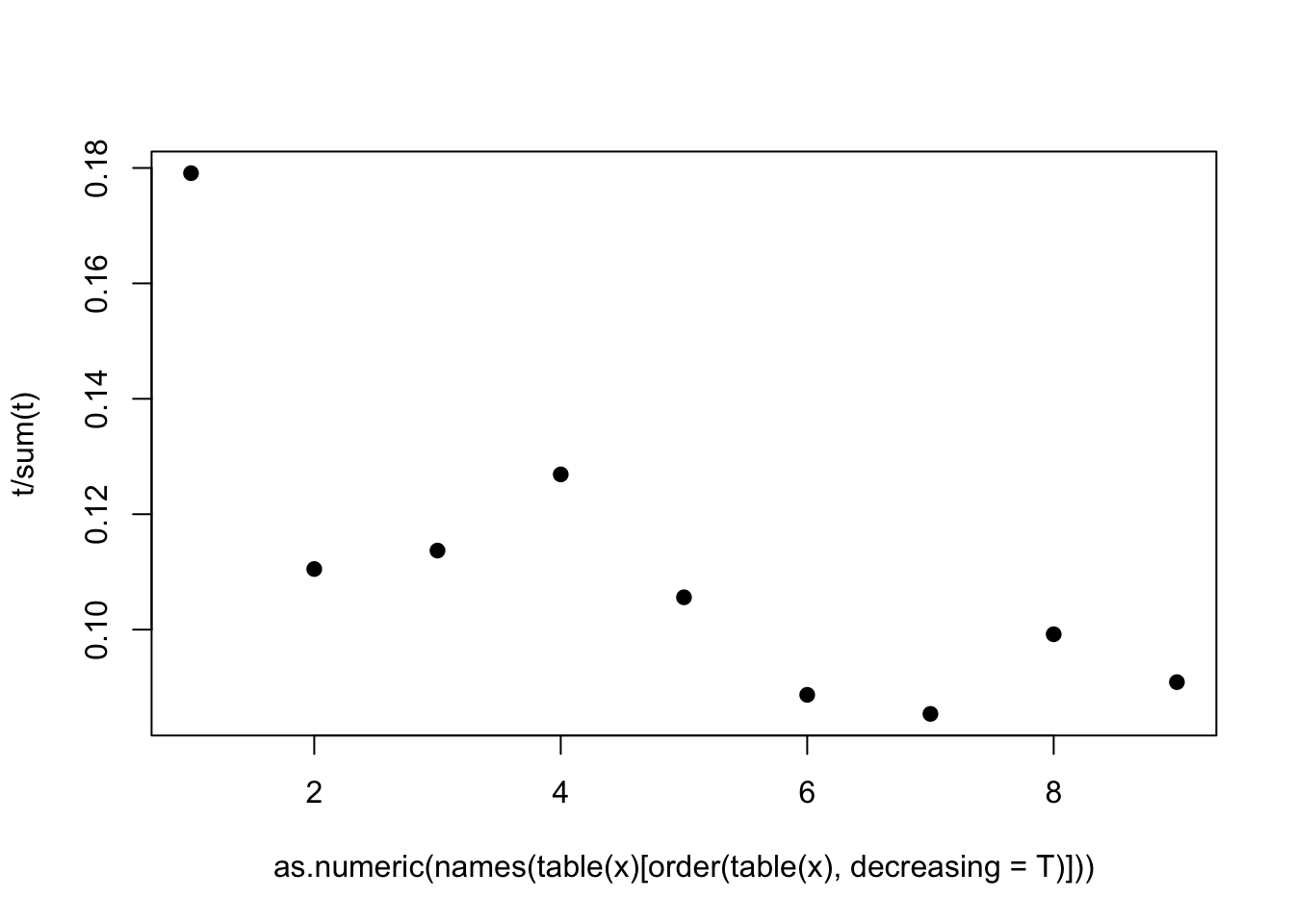3817 字

# 从真空里的球形鸡到社会财富分配（下）

$f(E) = A e^{-E/E_c}$

## 二八法则

string <- sample(c(LETTERS[1:10]," "),size = 100000,prob = c(rep(1/12,10),1/6),replace = T)
stringl <- paste(string, sep="", collapse="")
strings <- strsplit(stringl, " +")
t <- as.numeric(table(strings)[order(table(strings),decreasing = T)])
plot(log(t/sum(t))~log(c(1:length(t))),pch=19)## 本福德法则

t <- round(runif(10000,1,100000))
x <- c()
for(i in 1:10000){
}
t <- as.numeric(table(x)[order(table(x),decreasing = T)])
plot(t/sum(t)~as.numeric(names(table(x)[order(table(x),decreasing = T)])),pch=19)t <- round(runif(10000,1,100000)) + (1:9)^(round(runif(10000,1,9)))
## Warning in (1:9)^(round(runif(10000, 1, 9))): longer object length is not a
## multiple of shorter object length
x <- c()
for(i in 1:10000){
}
t <- as.numeric(table(x)[order(table(x),decreasing = T)])
plot(t/sum(t)~as.numeric(names(table(x)[order(table(x),decreasing = T)])),pch=19)